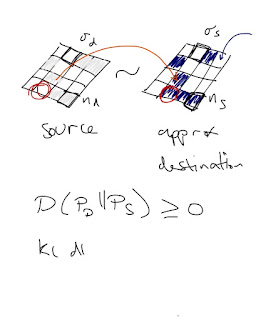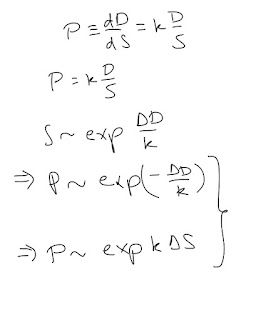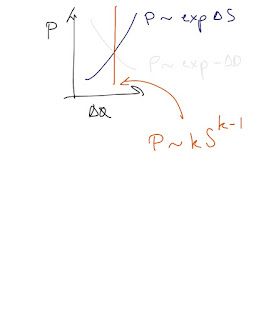## Saturday, October 24, 2015

### Info EQ 101

Also on my flight yesterday, I started writing up what I would say in a chalkboard lecture (or brown bag seminar) about information equilibrium.
Update: see the addendum for a bit more on some issues glossed over on the first segment relating to comments from Ken Duda below.

...At its heart, information equilibrium is about matching up probability distributions so that the probability distribution of demand matches up with the probability distribution of supply. More accurately, we'd say the information revealed by samples from one distribution is equal to the information revealed by samples from another. Let's say we have nd demand widgets on one board and ns supply widgets on another. The probability of a widget appearing on a square is 1/σ, so the information in revealing a widget on a square is - log 1/σ = log σ. The information in n of those widgets is n log σ.

Let's say the information in the two boards is equal so that nd log σd = ns log σs. Take the number of demand widgets to be large so that a single widget is an infinitesimal dD; in that case we can write nd = D/dD and ns = S/dS.

...

Let's substitute these new infinitesimal relationships and rearrange to form a differential equation. Let's call the ratio of the information due to the number of board positions log σd/log σs the information transfer index k.

We say the derivative defines an abstract price P.

Note the key properties of this equation: it's a marginal relationship and it satisfies homogeneity of degree zero.

We'll call this an information equilibrium relationship and use the notation P : D ⇄ S.

...Note that the distributions on our boards don't exactly have to match up. But you don't sell a widget if there's no demand and you don't sell as many widgets as you can (with no wasted widgets) unless you match the supply distribution with the demand distribution.

We can call the demand distribution the source distribution, or information source and the supply distribution the destination distribution. It functions as an approximation to the Platonic source distribution.

You could measure the information loss using the Kullback-Liebler divergence. However, information loss has a consequence for our differential equation.

...Since the information in the source is the best a destination (receiver) can receive, the information in the demand distribution is in general greater than the information in the supply distribution (or more technically it takes extra bits to decode a D signal using S than it does using D). When these differ, we call this non-ideal information transfer.

Non-ideal information transfer changes our differential equation into a differential inequality.

Which means (via Gronwall's inequality) that our solutions to the Diff Eq are just bounds on the non-ideal case.

What are those solutions?

...The first solution is where you take both supply and demand to vary together. This corresponds to general equilibrium. The solution is just a power law.

If we say our supply variables is an exponential as a function of time with supply growth rate σ, then demand and price are also exponentials with growth rates δ ~ k σ and π ~ (k - 1) σ, respectively.

...There are two other solutions we can get out of this equation. If we take supply to adjust more quickly than demand when it deviates from some initial value D0 (representing partial equilibrium in economics -- in thermodynamics, we'd say were in contact with a "supply bath"), then we get a different exponential solution.  The same goes for a demand bath and supply adjusting slowly.

Use ΔS and ΔD for S - S0 and D - D0, respectively.

...If we relate our partial equilibrium solutions to the definition of price we come up with relationships that give us supply and demand curves.

These should be interpreted in terms of price changes (the are shifts along the supply and demand curves). If price goes down, demand goes up. If price goes up, supply goes up.

Shifts of the curves involve changing the values of D0 and S0.

...From this we recover the basic Marshallian supply and demand diagram with information transfer index k relating to the price elasticities.

Our general solution also appears on this graph, but for that one ΔS = 0 and ΔD = 0 since we're in general, not partial equilibrium. We'll relate this to the long run aggregate supply curve in a minute.

...Note that if we have non-ideal information transfer, these solutions all become bounds on the market price, so the price can appear anywhere in this orange triangle.

If we take information equilibrium to hold approximately, we could get a price path (green) that has a bound (black). Normal growth here is punctuated by both a bout of more serious non-ideal information transfer (a recession?) and then a fast (and brief) change supply or demand (a big discovery of oil or a carbon tax, respectively).

...Since we really haven't specified anything about the widgets, we could easily take these to be aggregate demand widgets and aggregate supply widgets and P to be the price level.

We have the same solutions to the info eq diff eq again, with the supply curve representing the short run aggregate supple (SRAS) curve and the general equilibrium solution representing the long run aggregate supply (LRAS) curve.

...What if we have a more realistic system where aggregate demand is in information equilibrium with money and money is in info eq with aggregate supply?

Using the chain rule, we can show that the model is encompassed in a new information equilibrium relationship holds between AD and money (the AS relationship drops out in equilibrium) with a new information transfer index.

And we have the same general eq solution to the information equilibrium condition where AD grows with the money supply and so does the price level.

A generic "quantity theory of money"

...Let's say the money supply grows exponentially (as we did earlier) at a rate μ, inflation (price level growth) is π and nominal (AD) growth is ν.

Then π ~ (k - 1) μ and ν ~ k μ

Note that if k = 2, inflation equals the money supply growth rate.

What else?

...Let's say nominal growth is ν = ρ + π, where ρ is real growth and look at the ratio ν/π and write it in terms of the information transfer index and the growth rate of the money supply (which drops out).

If k is very large, then ν ≈ π, which implies that real growth ρ is small compared to inflation. That means large information transfer index is a high inflation limit.

Conversely, if the information transfer index is about 1, then the price level is roughly constant (the time dependence drops out to leading order). A low inflation limit.

1.I'm trying to figure out why a board with N widgets has N log \sigma units of information. As a software guy, it's easiest for me to think in bits, i.e. \sigma=2. If I have a word with 100 bits, then on average, that word has 50 1 bits e.g. 50 log 2 units of information. This makes sense to me except for the "on average" part. I don't see why a word with slightly more than 50 1 bits should be considered to have "more information" than a word with slightly less than 50 1 bits. I can accept that the amount of information depends on the number of bits that turns up 1; it's the asymmetry where extra 1 bits gives you more information and less-than-expected 1 bits gives you less that I'm finding, let's just say, unintuitive.

I am continuing to feel like I understand the ITM at a high level (I especially like your comment that incentives don't induce behavior, but rather open up additional state space for people to wander into) ... but I fail to grasp even the most basic ITM mathematical models. Which is frustrating to me, because I have no problem with Taylor series or the Master Method of solving recurrences or the Burnside counting lemma or whatever, I feel like this should be within reach.

Thanks,
-Ken

1.Hi Ken,

I appreciate your questions because it helps me improve my explanations ...

One thing to keep in mind is that I'm not just comparing the on bits, but also the off bits. So I should say revealing a widget on a square gives you log2 σ bits of information, but so does revealing a lack of a widget on a square.

I turn the above diagram into a probability distribution in this post:

http://informationtransfereconomics.blogspot.com/2015/05/the-economic-allocation-problem.html

The boards can be of different sizes -- the illustrations are essentially k = 1.

One way to think about these pictures is as a probability distribution: the probability density is high where there is a colored square and low where there isn't. It's not as intuitive with single bits, but in the link above I integrate along one of the axes and the probability distribution interpretation is more obvious.

2.Another thing -- part of why you need nd, ns >> 1 -- is so that the distribution of "on" bits are approximately equal to their probability distribution.

3.Jason, thanks as always for your patience.

> One thing to keep in mind is that I'm not just comparing
> the on bits, but also the off bits. So I should say revealing
> a widget on a square gives you log2 σ bits of information,
> but so does revealing a lack of a widget on a square.

Thanks, that clears it up completely in the case \sigma = 2.

However it creates additional confusion. If I have, say, \sigma = 100, then there is log 100 information in each square. But, I could equivalently take \sigma = 100/99, because I'm just inverting my sense of "on" and "off". Intuitively, swapping every "on" for an "off" should not change the amount of information. But, in my understanding of your formulation, the amount of information per square drops from log 100 to log 100/99 if I swap "on" and "off".

I think what's inconsistent here is whether \sigma is the reciprocal of the probability of finding a widget on the square, or is \sigma the number of different widgets that could occupy the square. From what I remember in some coding theory class I took, \sigma is generally the alphabet (or size of the alphabet) so I'm guessing that taking \sigma=100 for a system with two possible values in each square is just nonsense. But it would help if your text defined \sigma that way.

Thanks,
-Ken

4.I think I made a mistake in the previous comment that is due to the same confusion of probability and number of widgets. That's what I get for trying to answer comments with a cold. Let's see if I can set it straight.

The σ is the number of squares on the boards. There are 16 in the boards above, so imagine rolling a 16-sided die (uniform distribution) to place a given widget. Now σ = 16, so - log 1/σ = log 16.

Now n is the number of times you roll the die. When you roll a die, you mark a square. That means more than one widget can appear on a square. And the amount of information is n log σ.

In the above pictures, I only "rolled" about 6 times, so it is unlikely that more than one widget will appear on a square. The information is 6 log 16.

If n >> 1, say, 1024, then there should be on average 64 widgets on each square (± 2 at 1-sigma error). Now n >> 1 is where the distribution of widgets approximates the (uniform) probability distribution (it doesn't when n = 6).

The problem with my previous comment where the zeros matter is that I was essentially taking a board position as a set of on/off bits. In that case the board has σ bits of information regardless of the state of the widgets (colored squares). This problematic interpretation is actually encouraged by the way the diagrams are drawn, so I need to come up with a better version.

2.See here for a better (and illustrated) version of the response to Ken's comments above: